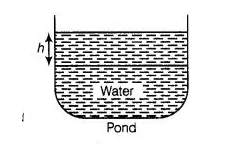# 500 persons are taking a dip into a cuboidal

Question:

500 persons are taking a dip into a cuboidal pond which is 80 m long and 50 m broad. What is the rise of water level in the pond, if the average

displacement of the water by a person is 0.04 m3?

Solution:

Let the rise of water level in the pond be hm, when 500 persons are taking a dip into a cuboidal pond.

Given thatLength of the cuboidal pond $=80 \mathrm{~m}$

Breadth of the cuboidal pond $=50 \mathrm{~m}$

Now, volume for the rise of water level in the pond

$=$ Length $\times$ Breadth $\times$ Height

$=80 \times 50 \times h=4000 h \mathrm{~m}^{3}$

and the average displacement of the water by a person $=0.04$

$\mathrm{~m}^{3}$

So, the average displacement of the water by 500 persons $=500 \times 0.04 \mathrm{~m}^{3}$

Now, by given condition,

Volume for the rise of water level in the pond = Average displacement of the water by

500 persons

$\Rightarrow$ $4000 h=500 \times 0.04$

$\therefore$ $h=\frac{500 \times 0.04}{4000}=\frac{20}{4000}=\frac{1}{200} \mathrm{~m}$

$=0.005 \mathrm{~m}$

$=0.005 \times 100 \mathrm{~cm}$

$=0.5 \mathrm{~cm}$

Hence, the required rise of water level in the pond is 0.5 cm.